If you have a related question, please click the "Ask a related question" button in the top right corner. The newly created question will be automatically linked to this question.

# LM318: Input impedance of inverting amplifier circuit

Part Number: LM318

Recently, I was looking at the calculation of the input impedance of the op-amp

This article describes in detail how to calculate the input impedance of the inverting amplifier circuit, not just the RIN，

But how to understand v+ and v-, in the article , the v- is vtest, v+=itest*Ra, I do not know how to get this formula, look forward to the expert's detailed answer.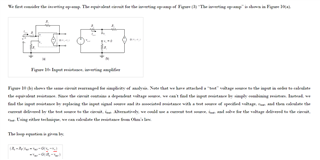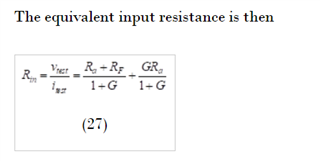www.tina.com/.../

• Hello,

The equation is simplified via substituting v+ and v- into equation 26.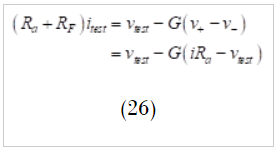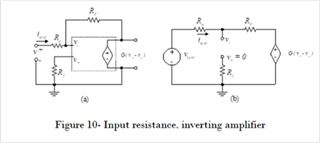Figure 10 notes that the V+ node of the inverting amplifier is equal to 0V. To solve for V-, start from ground in figure 10 and sum the voltage rise from Vtest with the voltage drop due to the current flowing through Ra: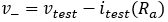Therefore: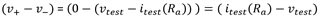Lastly, substitute (v+ - v-) back into the original equation: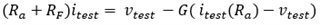Let me know if there is any confusion in solving for the equation.

Regards,

Jacob